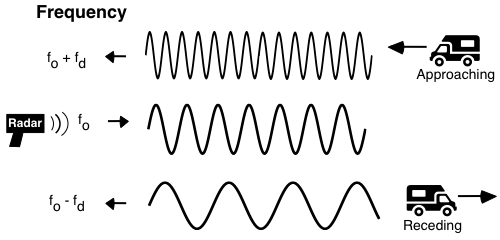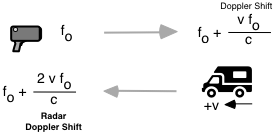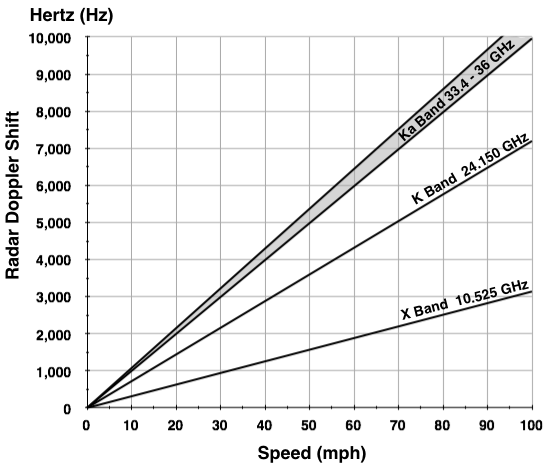Previous | Next

Police microwave radars all use the Doppler Principle to measure speed. The radar transmits a continuous microwave signal and simultaneously measures the echo that is frequency shifted proportional to speed, the Doppler Shift. The frequency shift is measured in cycles per second and has unit dimensions of Hertz (Hz).

The horn from a moving train is a good example of the Doppler effect. As the train approaches a stationary listener the frequency pitch of the whistle sounds higher than when the train is even with the listener. As the train recedes from the listener the pitch decreases. Car horns exhibit the same phenomenon, as does all sound.

In the above example if a car horn is stationary and a listener is on the train the Doppler principle still applies. As the listener on the train approaches the stationary horn the pitch of the horn sounds higher, as the train recedes from the stationary horn the pitch sounds lower to anyone on the train.

Microwave signals travel at the speed of light but still obey the Doppler Principle. Microwave radars receive a Doppler frequency shifted reflection from a moving object. Frequency is shifted higher for approaching objects, and lower for receding objects. The frequency shift is proportional to speed.

Echo Frequency Shift
Approaching Traffic Receding Traffic
ft = fo + fd ft = fo - fd

ft = Traffic Echo (return) Frequency
fo = Transmit FrequencyRadar Doppler shift (fd) is a function of transmit frequency (fo), speed of wave (c = speed of light), and target vehicle speed (v). Speed is positive (+v) for approaching traffic and negative (-v) for receding traffic.fd = Radar Doppler Shift fo = Transmit Frequency v = vehicle speed c = Speed of Light

 fo = Radar Frequency: GHz vo = Target Vehicle Speed: mph Radar Doppler Shift in Herts (Hz)Measured Speed
v = fd c / (2 fo)
 v = Traffic Speed (-v for receding traffic) fd = Radar Doppler Shift (-fd for receding traffic) fo = Transmit Frequency c = Speed of Light

Radar Doppler Shift at Police Frequencies
The table below shows radar Doppler shift per speed unit (1 unit) for common police radar frequencies. Radar Doppler shift is unit shift multiplied by speed. For example an X band radar Doppler shift for a 100 mph vehicle is 31.39 Hz/mph multiplied by 100 mph and equals 3,139 Hertz.

Unit Speed Doppler Shift

 Radar Transmit Frequency Radar Doppler Shift Band GHz Hz/kph Hz/mph Hz/knot S 2.455 4.53 7.29 8.39 X 9.410 17.44 28.06 32.30 X 9.900 18.34 29.53 33.98 X 10.525 19.50 31.39 36.12 Ku 13.450 24.92 40.11 46.16 K 24.125 44.71 71.95 82.80 K 24.150 44.75 72.02 82.88 Ka 33.4 -36.0 61.89 -66.71 99.61 -107.36 114.63 -123.55Frequency Spectrum
The transmit signal has some noise skirts that interfere with echoes from slow moving objects. Most radars have a minimum speed specification, typically 10 - 15 mph minimum target speed. A few radars have a 1 - 3 mph minimum speed.